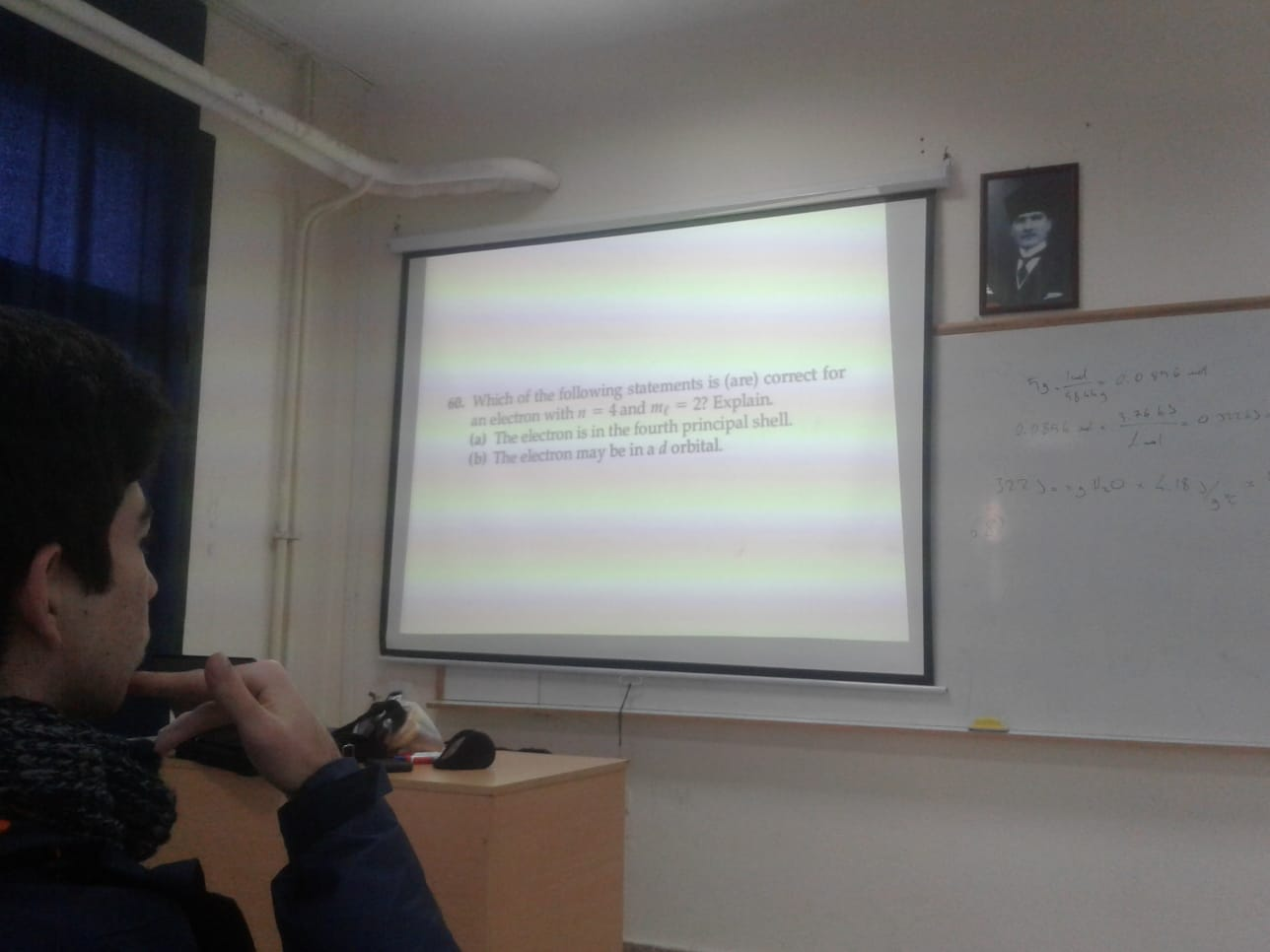# 6. Which of the following statements is (are) correct foran electron with n = 4 and m= 22 Explain.(a) The electron is in the fourth principal shell.(b) The electron may be in a d orbital.53.3.26 4S0.055L

Question
6 viewshelp_outlineImage Transcriptionclose6. Which of the following statements is (are) correct for an electron with n = 4 and m= 22 Explain. (a) The electron is in the fourth principal shell. (b) The electron may be in a d orbital. 53. 3.26 4S 0.055L fullscreen
check_circle

Step 1

An atom is mainly composed of three sub-particles; electrons, protons and neutrons. These particles are arranged in a certain way in the atom and provide electrical neutrality to the molecule. Electrons are placed in different energy levels of an atom.

Step 2

The energy levels of electrons in an atom can be explained with the help of different quantum numbers.

• Principal quantum number ‘n’- It represents the number of shell in an atom. It defines the size of atom and also related to another quantum number ‘l’.
• Azimuthal quantum number ‘l’ - It represents the number of sub-shell in an atom. The values of ‘l’ can be from 0 to n-1. Here 0 is for s-subshell, 1 is for p-subshell, 2 is for d-subshell and 3 is for f-subshell.
• Magnetic quantum number ‘ml’- It represents the number of orbitals and can be from -l to +l including 0.
• Spin quantum number- It indicates the spin of electrons and can be +1/2 or -1/2.
Step 3

Hence n = 4 indicates that 4 must be the shell number. Th...

### Want to see the full answer?

See Solution

#### Want to see this answer and more?

Solutions are written by subject experts who are available 24/7. Questions are typically answered within 1 hour.*

See Solution
*Response times may vary by subject and question.
Tagged in

### Atomic structure